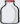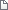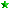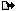Solving equations card game
mgravesPosted 3/2/2012 9:03 AM (#3366) Subject: Solving equations card game
Math

Posts: 42Location: Bryan Station
Solving equations card game ( alg 1) Students must find cards in order to solve each equation

Attachments
----------------equation card game A.docx (36KB - 489 downloads)Linear equation card game B.docx (31KB - 441 downloads)Linear equation card game C.docx (45KB - 399 downloads)